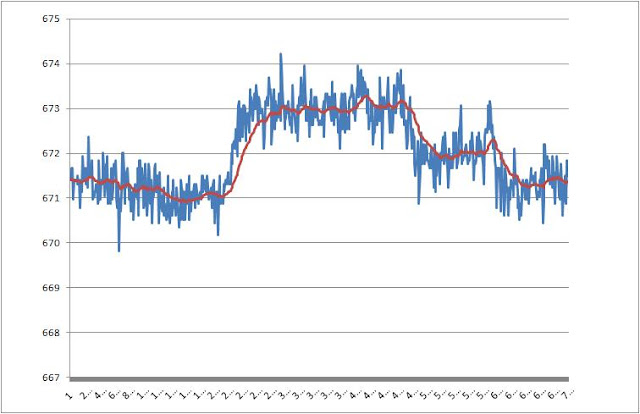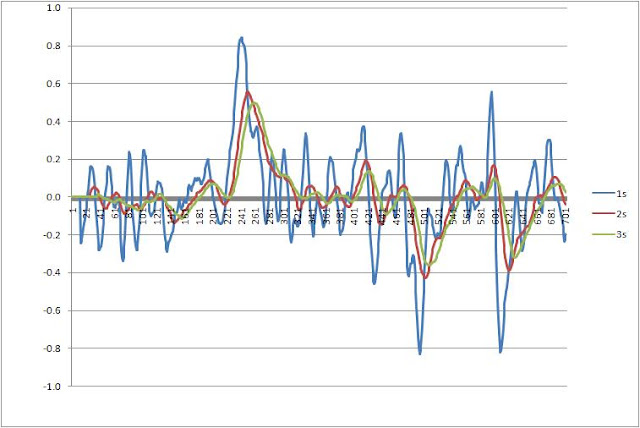## Sunday, 22 May 2011

### Processing and filtering the data

A raw stream of pressure data is noisy. If it was displayed directly to the user as altitude it would not mean much as it jumps around and causes more confusion than good. For a useful altimeter we need to convert the pressure to altitude and smooth the data out. For the vario we need to determine the rate of change of altitude.

Pressure to altitude conversion

The BMP085 tutorial on Sparkfun provides a handy reference to the pressure to altitude conversion. The altitude equation near the bottom of the tutorial is the one to use. Read more about QNH here to understand all about the relationship between pressure and altitude.

Filtering the altitude data

The raw stream of pressure data converted to altitude is shown in the image below. To get this data the alpha prototype was kept at constant altitude for about 20s, then lifted up about 2m and kept there for 20s, then down 1m and constant for 10s, then back to the original height for 10s.

To turn the noisy data into a smooth line we need to filter the data. The trick is to balance the degree of filtering, which is really just different ways to sum up and balance historical data, with the degree of lag you are willing to accept. For the altimeter we can accept seconds of lag and there is no real issue, up to about 10 seconds is fine. This means that we can use a well damped filter.

There are a bunch of choices. A simple average is perhaps what most people might instinctively use. Just by averaging the last 20 or 40 measurements (representing one or two seconds) you will get a much smoother line. The problem with doing the maths is a little more complex than needed as you require to save each of the last x measurements then do the averaging math each time you need to display the altitude. This is not an issue on a PC or Android device, but is a pain on a micro controller.

A more simple approach is to use an IIR filter. This wikipedia article will confuse the hell out of most people. It is actually really easy when explained in words. You measure the altitude the first time. This becomes the 'current' altitude. With each subsequent measurement you sum X% of the new measurement with (100 - X)% of the previous 'current' altitude for the new 'current' altitude. This ends up being an exponential filter, where the most recent measurements have more weight than older measurements. The X% is the damping factor. Around 5 or 10 is about right, the lower the number the more damping. More damping equals more smoothness but more lag.

A more complex approach is to use a one dimensional Kalman filter. After playing with this I managed to work out that it is pretty much equivalent to the IIR filter, once it has been tweaked. I am going to use an IIR filter for altitude.

Linear regression for vertical speed

To turn the altitude data to vertical speed we need to determine the slope of the graph. The easiest way to do this is to take a linear regression over a time window. Again, the greater the window the more smoothing, but the greater the lag. In the bottom image I show how 1, 2 and 3 seconds regression windows work. 1s is too noisy. You can see that in the first 20s there are many spurious vario blips over 0.1 m/s. 2s and 3s are much better (and about the same). You can see at the 20s mark when the vario was lifted the vertical speed up around 0.5 m/s. There is a little more lag on the 3s data window compared to the 2s window as expected.

You don't need to the the entire linear regression, just calculate the slope. I have a working vario!Raw pressure data converted to altitude (blue) with IIR filter (red - damping factor 5%)Linear regression of pressure data over various time periods